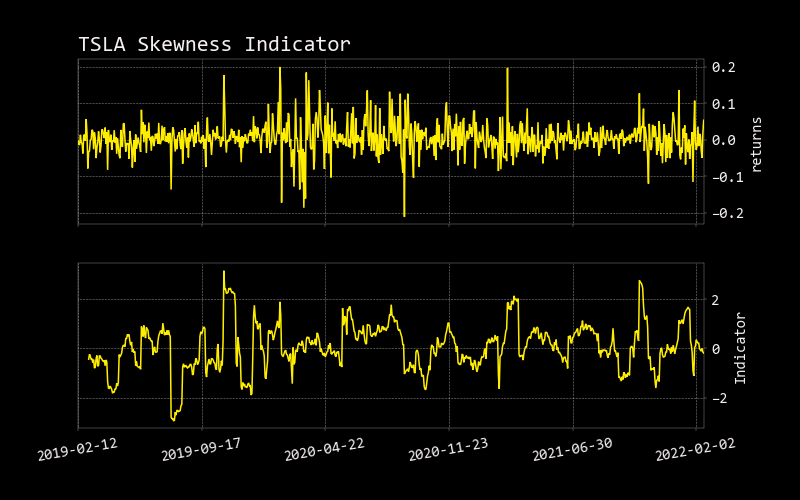# Skew

``````usage: skew [-l N_LENGTH] [--export {csv,json,xlsx}] [-h]
``````

Skewness is a measure of asymmetry or distortion of symmetric distribution. It measures the deviation of the given distribution of a random variable from a symmetric distribution, such as normal distribution. A normal distribution is without any skewness, as it is symmetrical on both sides. Hence, a curve is regarded as skewed if it is shifted towards the right or the left.

See the Wiki page for a detailed explanation: https://en.wikipedia.org/wiki/Skewness

``````optional arguments:
-l N_LENGTH, --length N_LENGTH
length (default: 14)
--export {csv,json,xlsx}
Export dataframe data to csv,json,xlsx file (default: )
-h, --help            show this help message (default: False)
``````